# B. Nikolic - Renormalizability of the D-Deformed Wess-Zumino Model

Sep. 11, 2011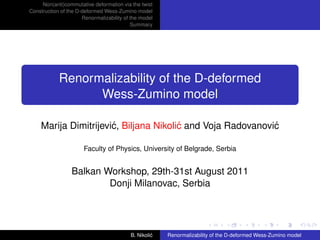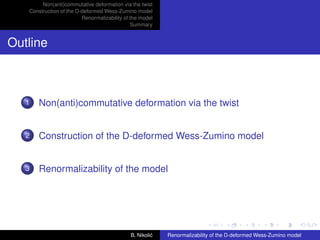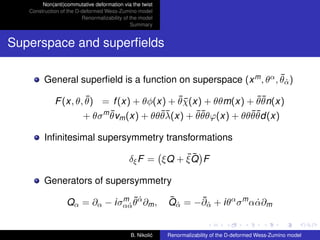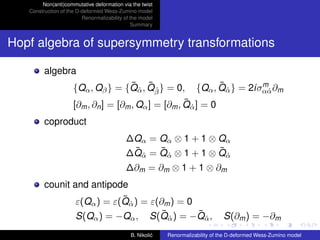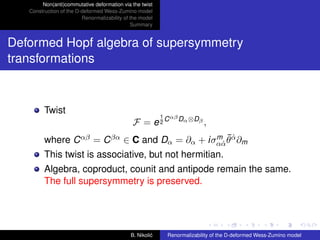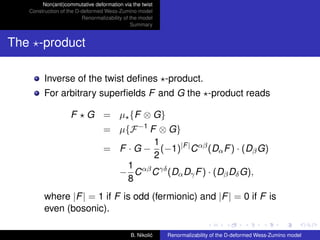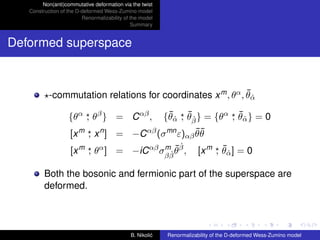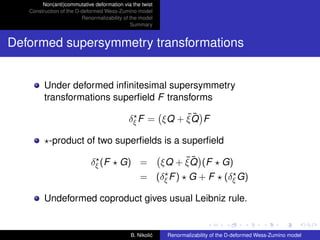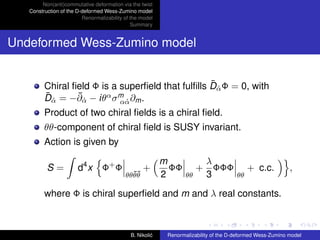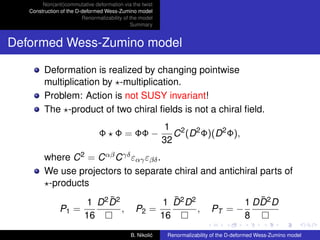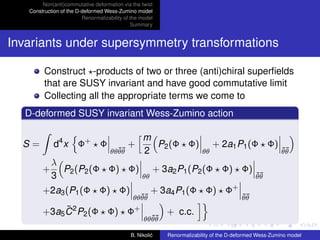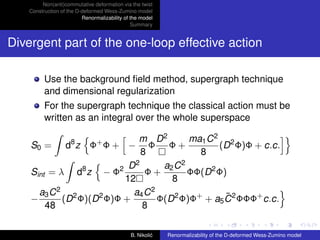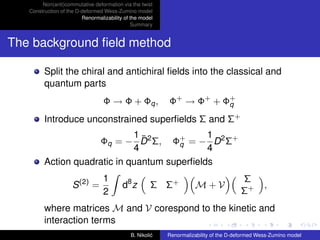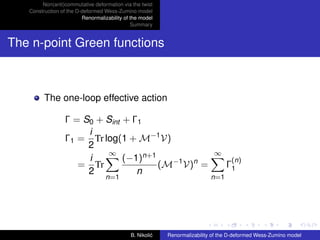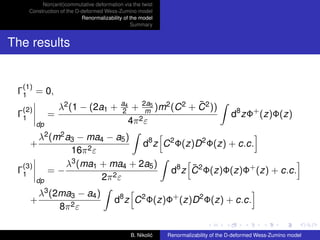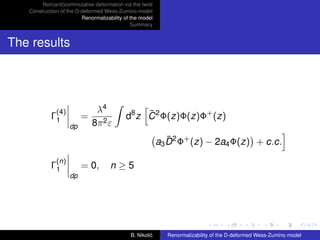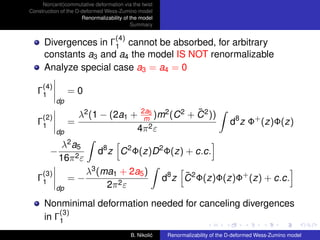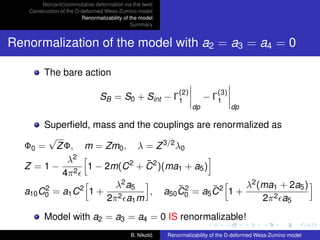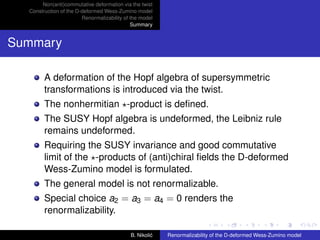1 of 19

### B. Nikolic - Renormalizability of the D-Deformed Wess-Zumino Model

• 1. Non(anti)commutative deformation via the twist Construction of the D-deformed Wess-Zumino model Renormalizability of the model Summary Renormalizability of the D-deformed Wess-Zumino model Marija Dimitrijevi´ , Biljana Nikoli´ and Voja Radovanovi´ c c c Faculty of Physics, University of Belgrade, Serbia Balkan Workshop, 29th-31st August 2011 Donji Milanovac, Serbia B. Nikoli´ c Renormalizability of the D-deformed Wess-Zumino model
• 2. Non(anti)commutative deformation via the twist Construction of the D-deformed Wess-Zumino model Renormalizability of the model Summary Outline 1 Non(anti)commutative deformation via the twist 2 Construction of the D-deformed Wess-Zumino model 3 Renormalizability of the model B. Nikoli´ c Renormalizability of the D-deformed Wess-Zumino model
• 3. Non(anti)commutative deformation via the twist Construction of the D-deformed Wess-Zumino model Renormalizability of the model Summary Superspace and superﬁelds ¯˙ General superﬁeld is a function on superspace (x m , θα , θα ) ¯ ¯¯ ¯¯ F (x, θ, θ) = f (x) + θφ(x) + θχ(x) + θθm(x) + θθn(x) ¯ ¯¯ ¯¯ ¯¯ + θσ m θvm (x) + θθθλ(x) + θθθϕ(x) + θθθθd(x) Inﬁnitesimal supersymmetry transformations ¯¯ δξ F = ξQ + ξ Q F Generators of supersymmetry m ¯˙ Qα = ∂α − iσαα θα ∂m , ¯˙ ¯˙ Qα = −∂α + iθα σ m αα∂m ˙ ˙ B. Nikoli´ c Renormalizability of the D-deformed Wess-Zumino model
• 4. Non(anti)commutative deformation via the twist Construction of the D-deformed Wess-Zumino model Renormalizability of the model Summary Hopf algebra of supersymmetry transformations algebra ¯˙ ¯˙ ¯˙ m {Qα , Qβ } = {Qα , Qβ } = 0, {Qα , Qα } = 2iσαα ∂m ˙ ¯ α] = 0 [∂m , ∂n ] = [∂m , Qα ] = [∂m , Q ˙ coproduct ∆Qα = Qα ⊗ 1 + 1 ⊗ Qα ¯˙ ¯˙ ¯˙ ∆Qα = Qα ⊗ 1 + 1 ⊗ Qα ∆∂m = ∂m ⊗ 1 + 1 ⊗ ∂m counit and antipode ¯˙ ε(Qα ) = ε(Qα ) = ε(∂m ) = 0 S(Qα ) = −Qα , ¯˙ ¯˙ S(Qα ) = −Qα , S(∂m ) = −∂m B. Nikoli´ c Renormalizability of the D-deformed Wess-Zumino model
• 5. Non(anti)commutative deformation via the twist Construction of the D-deformed Wess-Zumino model Renormalizability of the model Summary Deformed Hopf algebra of supersymmetry transformations Twist 1 αβ D ⊗D F = e2C α β , m ¯˙ where C αβ = C βα ∈ C and Dα = ∂α + iσαα θα ∂m ˙ This twist is associative, but not hermitian. Algebra, coproduct, counit and antipode remain the same. The full supersymmetry is preserved. B. Nikoli´ c Renormalizability of the D-deformed Wess-Zumino model
• 6. Non(anti)commutative deformation via the twist Construction of the D-deformed Wess-Zumino model Renormalizability of the model Summary The -product Inverse of the twist deﬁnes -product. For arbitrary superﬁelds F and G the -product reads F G = µ {F ⊗ G} = µ{F −1 F ⊗ G} 1 = F · G − (−1)|F | C αβ (Dα F ) · (Dβ G) 2 1 αβ γδ − C C (Dα Dγ F ) · (Dβ Dδ G), 8 where |F | = 1 if F is odd (fermionic) and |F | = 0 if F is even (bosonic). B. Nikoli´ c Renormalizability of the D-deformed Wess-Zumino model
• 7. Non(anti)commutative deformation via the twist Construction of the D-deformed Wess-Zumino model Renormalizability of the model Summary Deformed superspace ¯˙ -commutation relations for coordinates x m , θα , θα {θα , θβ } = C αβ , ¯˙ ¯ ˙ ¯˙ {θα , θβ } = {θα , θα } = 0 ¯¯ [x m , x n ] = −C αβ (σ mn ε)αβ θθ m ¯ ˙ ¯˙ [x m , θα ] = −iC αβ σβ β θβ , ˙ [x m , θα ] = 0 Both the bosonic and fermionic part of the superspace are deformed. B. Nikoli´ c Renormalizability of the D-deformed Wess-Zumino model
• 8. Non(anti)commutative deformation via the twist Construction of the D-deformed Wess-Zumino model Renormalizability of the model Summary Deformed supersymmetry transformations Under deformed inﬁnitesimal supersymmetry transformations superﬁeld F transforms ¯¯ δξ F = ξQ + ξ Q F -product of two superﬁelds is a superﬁeld δξ (F G) = ¯¯ ξQ + ξ Q (F G) = (δξ F ) G + F (δξ G) Undeformed coproduct gives usual Leibniz rule. B. Nikoli´ c Renormalizability of the D-deformed Wess-Zumino model
• 9. Non(anti)commutative deformation via the twist Construction of the D-deformed Wess-Zumino model Renormalizability of the model Summary Undeformed Wess-Zumino model ¯˙ Chiral ﬁeld Φ is a superﬁeld that fulﬁlls Dα Φ = 0, with ¯ α = −∂α − iθα σ m ∂m . D˙ ¯˙ αα ˙ Product of two chiral ﬁelds is a chiral ﬁeld. θθ-component of chiral ﬁeld is SUSY invariant. Action is given by m λ S= d4 x Φ+ Φ + ΦΦ + ΦΦΦ + c.c. , ¯¯ θθθθ 2 θθ 3 θθ where Φ is chiral superﬁeld and m and λ real constants. B. Nikoli´ c Renormalizability of the D-deformed Wess-Zumino model
• 10. Non(anti)commutative deformation via the twist Construction of the D-deformed Wess-Zumino model Renormalizability of the model Summary Deformed Wess-Zumino model Deformation is realized by changing pointwise multiplication by -multiplication. Problem: Action is not SUSY invariant! The -product of two chiral ﬁelds is not a chiral ﬁeld. 1 2 2 Φ Φ = ΦΦ − C (D Φ)(D 2 Φ), 32 where C 2 = C αβ C γδ εαγ εβδ . We use projectors to separate chiral and antichiral parts of -products ¯ 1 D2D2 ¯ 1 D2D2 ¯ 1 DD2D P1 = , P2 = , PT = − 16 16 8 B. Nikoli´ c Renormalizability of the D-deformed Wess-Zumino model
• 11. Non(anti)commutative deformation via the twist Construction of the D-deformed Wess-Zumino model Renormalizability of the model Summary Invariants under supersymmetry transformations Construct -products of two or three (anti)chiral superﬁelds that are SUSY invariant and have good commutative limit Collecting all the appropriate terms we come to D-deformed SUSY invariant Wess-Zumino action m S= d4 x Φ+ Φ + P2 (Φ Φ) + 2a1 P1 (Φ Φ) ¯¯ θθθθ 2 θθ ¯¯ θθ λ + P2 (P2 (Φ Φ) Φ) + 3a2 P1 (P2 (Φ Φ) Φ) 3 θθ ¯¯ θθ + +2a3 (P1 (Φ Φ) Φ) + 3a4 P1 (Φ Φ) Φ ¯¯ θθθθ ¯¯ θθ ¯2 +3a5 C P2 (Φ Φ) Φ + + c.c. ¯¯ θθθθ B. Nikoli´ c Renormalizability of the D-deformed Wess-Zumino model
• 12. Non(anti)commutative deformation via the twist Construction of the D-deformed Wess-Zumino model Renormalizability of the model Summary Divergent part of the one-loop effective action Use the background ﬁeld method, supergraph technique and dimensional regularization For the supergraph technique the classical action must be written as an integral over the whole superspace m D2 ma1 C 2 2 S0 = d8 z Φ+ Φ + −Φ Φ+ (D Φ)Φ + c.c. 8 8 D2 a2 C 2 Sint = λ d8 z − Φ2 Φ+ ΦΦ(D 2 Φ) 12 8 a3 C 2 2 a4 C 2 ¯ − (D Φ)(D 2 Φ)Φ + Φ(D 2 Φ)Φ+ + a5 C 2 ΦΦΦ+ c.c. 48 8 B. Nikoli´ c Renormalizability of the D-deformed Wess-Zumino model
• 13. Non(anti)commutative deformation via the twist Construction of the D-deformed Wess-Zumino model Renormalizability of the model Summary The background ﬁeld method Split the chiral and antichiral ﬁelds into the classical and quantum parts Φ → Φ + Φq , Φ+ → Φ+ + Φ+ q Introduce unconstrained superﬁelds Σ and Σ+ 1¯ 1 Φq = − D 2 Σ, Φ+ = − D 2 Σ+ q 4 4 Action quadratic in quantum superﬁelds 1 Σ S (2) = d8 z Σ Σ+ M+V , 2 Σ+ where matrices M and V corespond to the kinetic and interaction terms B. Nikoli´ c Renormalizability of the D-deformed Wess-Zumino model
• 14. Non(anti)commutative deformation via the twist Construction of the D-deformed Wess-Zumino model Renormalizability of the model Summary The n-point Green functions The one-loop effective action Γ = S0 + Sint + Γ1 i Γ1 = Tr log(1 + M−1 V) 2 ∞ ∞ i (−1)n+1 (n) = Tr (M−1 V)n = Γ1 2 n n=1 n=1 B. Nikoli´ c Renormalizability of the D-deformed Wess-Zumino model
• 15. Non(anti)commutative deformation via the twist Construction of the D-deformed Wess-Zumino model Renormalizability of the model Summary The results (1) Γ1 = 0, λ2 (1 − (2a1 + a4 ¯ + 2a5 )m2 (C 2 + C 2 )) (2) Γ1 = 2 m d8 zΦ+ (z)Φ(z) dp 4π 2 ε λ2 (m2 a3 − ma4 − a5 ) + d8 z C 2 Φ(z)D 2 Φ(z) + c.c. 16π 2 ε (3) λ3 (ma1 + ma4 + 2a5 ) ¯ Γ1 =− 2ε d8 z C 2 Φ(z)Φ(z)Φ+ (z) + c.c. dp 2π λ3 (2ma3 − a4 ) + d8 z C 2 Φ(z)Φ+ (z)D 2 Φ(z) + c.c. 8π 2 ε B. Nikoli´ c Renormalizability of the D-deformed Wess-Zumino model
• 16. Non(anti)commutative deformation via the twist Construction of the D-deformed Wess-Zumino model Renormalizability of the model Summary The results (4) λ4 ¯ Γ1 = d8 z C 2 Φ(z)Φ(z)Φ+ (z) dp 8π 2 ε ¯ a3 D 2 Φ+ (z) − 2a4 Φ(z) + c.c. (n) Γ1 = 0, n≥5 dp B. Nikoli´ c Renormalizability of the D-deformed Wess-Zumino model
• 17. Non(anti)commutative deformation via the twist Construction of the D-deformed Wess-Zumino model Renormalizability of the model Summary (4) Divergences in Γ1 cannot be absorbed, for arbitrary constants a3 and a4 the model IS NOT renormalizable Analyze special case a3 = a4 = 0 (4) Γ1 =0 dp ¯ λ2 (1 − (2a1 + 2a5 )m2 (C 2 + C 2 )) (2) Γ1 = m d8 z Φ+ (z)Φ(z) dp 4π 2 ε λ2 a5 − d8 z C 2 Φ(z)D 2 Φ(z) + c.c. 16π 2 ε (3) λ3 (ma1 + 2a5 ) ¯ Γ1 =− d8 z C 2 Φ(z)Φ(z)Φ+ (z) + c.c. dp 2π 2 ε Nonminimal deformation needed for canceling divergences (3) in Γ1 B. Nikoli´ c Renormalizability of the D-deformed Wess-Zumino model
• 18. Non(anti)commutative deformation via the twist Construction of the D-deformed Wess-Zumino model Renormalizability of the model Summary Renormalization of the model with a2 = a3 = a4 = 0 The bare action (2) (3) SB = S0 + Sint − Γ1 − Γ1 dp dp Superﬁeld, mass and the couplings are renormalized as √ Φ0 = Z Φ, m = Zm0 , λ = Z 3/2 λ0 λ2 ¯ Z = 1 − 2 1 − 2m(C 2 + C 2 )(ma1 + a5 ) 4π λ2 a5 ¯2 ¯ λ2 (ma1 + 2a5 ) a10 C0 = a1 C 2 1 + 2 2 , a50 C0 = a5 C 2 1 + 2π a1 m 2π 2 a5 Model with a2 = a3 = a4 = 0 IS renormalizable! B. Nikoli´ c Renormalizability of the D-deformed Wess-Zumino model
• 19. Non(anti)commutative deformation via the twist Construction of the D-deformed Wess-Zumino model Renormalizability of the model Summary Summary A deformation of the Hopf algebra of supersymmetric transformations is introduced via the twist. The nonhermitian -product is deﬁned. The SUSY Hopf algebra is undeformed, the Leibniz rule remains undeformed. Requiring the SUSY invariance and good commutative limit of the -products of (anti)chiral ﬁelds the D-deformed Wess-Zumino model is formulated. The general model is not renormalizable. Special choice a2 = a3 = a4 = 0 renders the renormalizability. B. Nikoli´ c Renormalizability of the D-deformed Wess-Zumino model